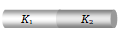The dimensions of thermal resistance are
(a) ${\mathrm{M}}^{-1}{\mathrm{L}}^{-2}{\mathrm{T}}^{3}\mathrm{K}$           (b) ${\mathrm{ML}}^{2}{\mathrm{T}}^{-2}{\mathrm{K}}^{-1}$
(c) ${\mathrm{ML}}^{2}{\mathrm{T}}^{-3}\mathrm{K}$               (d) ${\mathrm{ML}}^{2}{\mathrm{T}}^{-2}{\mathrm{K}}^{-2}$

Concept Questions :-

Conduction
High Yielding Test Series + Question Bank - NEET 2020

Difficulty Level:

Two walls of thicknesses ${\mathrm{d}}_{1}$ and ${\mathrm{d}}_{2}$ and thermal conductivities ${\mathrm{k}}_{1}$ and ${\mathrm{k}}_{2}$ are in contact. In the steady state, if the temperatures at the outer surfaces  are ${\mathrm{T}}_{1}$ and ${\mathrm{T}}_{2}$, the temperature at the common wall is  -

(a) $\frac{{\mathrm{k}}_{1}{\mathrm{T}}_{1}{\mathrm{d}}_{2}+{\mathrm{k}}_{2}{\mathrm{T}}_{2}{\mathrm{d}}_{1}}{{\mathrm{k}}_{1}{\mathrm{d}}_{2}+{\mathrm{k}}_{2}{\mathrm{d}}_{1}}$                      (b)$\frac{{\mathrm{k}}_{1}{\mathrm{T}}_{1}+{\mathrm{k}}_{2}{\mathrm{d}}_{2}}{{\mathrm{d}}_{1}+{\mathrm{d}}_{2}}$
(c) $\left(\frac{{\mathrm{k}}_{1}{\mathrm{d}}_{1}+{\mathrm{k}}_{2}{\mathrm{d}}_{2}}{{\mathrm{T}}_{1}+{\mathrm{T}}_{2}}\right){\mathrm{T}}_{1}{\mathrm{T}}_{2}$                    (d) $\frac{{\mathrm{k}}_{1}{\mathrm{d}}_{1}{\mathrm{T}}_{1}+{\mathrm{k}}_{2}{\mathrm{d}}_{2}{\mathrm{T}}_{2}}{{\mathrm{k}}_{1}{\mathrm{d}}_{1}+{\mathrm{k}}_{2}{\mathrm{d}}_{2}}$

Concept Questions :-

Conduction
High Yielding Test Series + Question Bank - NEET 2020

Difficulty Level:

A slab consists of two parallel layers of copper and brass of the same thickness and having thermal conductivities in the ratio 1 : 4. If the free face of brass is at 100°C and that of copper at 0°C, the temperature of interface is

(a) 80°C                (b) 20°C
(c) 60°C                (d) 40°C

Concept Questions :-

Conduction
High Yielding Test Series + Question Bank - NEET 2020

Difficulty Level:

Two thin blankets keep more hotness than one blanket of thickness equal to these two. The reason is

(a) Their surface area increases

(b) A layer of air is formed between these two blankets, which is bad conductor

(c) These have more wool

(d) They absorb more heat from outside

Concept Questions :-

Conduction

Difficulty Level:

Ice formed over lakes has

(a) Very high thermal conductivity and helps in further ice formation

(b) Very low conductivity and retards further formation of ice

(c) It permits quick convection and retards further formation of ice

(d) It is very good radiator

Concept Questions :-

Conduction

Difficulty Level:

Wires A and B have identical lengths and have circular cross-sections. The radius of A is twice the radius of B i.e. ${\mathrm{r}}_{\mathrm{A}}=2{\mathrm{r}}_{\mathrm{B}}$ . For a given temperature difference between the two ends, both wires conduct heat at the same rate. The relation between the thermal conductivities is given by

(a) ${\mathrm{K}}_{\mathrm{A}}=4{\mathrm{K}}_{\mathrm{B}}$                     (b) ${\mathrm{K}}_{\mathrm{A}}=2{\mathrm{K}}_{\mathrm{B}}$
(c) ${\mathrm{K}}_{\mathrm{A}}={\mathrm{K}}_{\mathrm{B}}/2$                   (d) ${\mathrm{K}}_{\mathrm{A}}={\mathrm{K}}_{\mathrm{B}}/4$

Concept Questions :-

Conduction
High Yielding Test Series + Question Bank - NEET 2020

Difficulty Level:

Two identical plates of different metals are joined to form a single plate whose thickness is double the thickness of each plate. If the coefficients of conductivity of each plate are 2 and 3 respectively, then the conductivity of composite plate will be
(a) 5                     (b) 2.4
(c) 1.5                  (d) 1.2

Concept Questions :-

Conduction
High Yielding Test Series + Question Bank - NEET 2020

Difficulty Level:

The coefficients of thermal conductivity of copper, mercury and glass are respectively ${\mathrm{K}}_{\mathrm{c}}$, ${\mathrm{K}}_{\mathrm{m}}$ and ${\mathrm{K}}_{\mathrm{g}}$ such that ${\mathrm{K}}_{\mathrm{c}}>{\mathrm{K}}_{\mathrm{m}}>{\mathrm{K}}_{\mathrm{g}}$. If the same quantity of heat is to flow per second per unit area of each and corresponding temperature gradients are ${\mathrm{X}}_{\mathrm{c}}$${\mathrm{X}}_{\mathrm{m}}$ and ${\mathrm{X}}_{\mathrm{g}}$, then
(a) ${\mathrm{X}}_{\mathrm{c}}={\mathrm{X}}_{\mathrm{m}}={\mathrm{X}}_{\mathrm{g}}$                  (b) ${\mathrm{X}}_{\mathrm{c}}>{\mathrm{X}}_{\mathrm{m}}>{\mathrm{X}}_{\mathrm{g}}$
(c) ${\mathrm{X}}_{\mathrm{c}}<{\mathrm{X}}_{\mathrm{m}}<{\mathrm{X}}_{\mathrm{g}}$                  (d) ${\mathrm{X}}_{\mathrm{m}}<{\mathrm{X}}_{\mathrm{c}}<{\mathrm{X}}_{\mathrm{g}}$

Concept Questions :-

Conduction
High Yielding Test Series + Question Bank - NEET 2020

Difficulty Level:

If two metallic plates of equal thicknesses and thermal conductivities ${\mathrm{K}}_{1}$ and ${\mathrm{K}}_{2}$ are put together face to face and a common plate is constructed, then the equivalent thermal conductivity of this plate will be(a) $\frac{{\mathrm{K}}_{1}{\mathrm{K}}_{2}}{{\mathrm{K}}_{1}+{\mathrm{K}}_{2}}$                     (b) $\frac{2{\mathrm{K}}_{1}{\mathrm{K}}_{2}}{{\mathrm{K}}_{1}+{\mathrm{K}}_{2}}$
(c) $\frac{{\left({\mathrm{K}}_{1}^{2}+{\mathrm{K}}_{2}^{2}\right)}^{3/2}}{{\mathrm{K}}_{1}{\mathrm{K}}_{2}}$              (d) $\frac{{\left({\mathrm{K}}_{1}^{2}+{\mathrm{K}}_{2}^{2}\right)}^{3/2}}{2{\mathrm{K}}_{1}{\mathrm{K}}_{2}}$

Concept Questions :-

Conduction
High Yielding Test Series + Question Bank - NEET 2020

Difficulty Level:

The quantity of heat which crosses unit area of a metal plate during conduction depends upon

(a) The density of the metal

(b) The temperature gradient perpendicular to the area

(c) The temperature to which the metal is heated

(d) The area of the metal plate

Concept Questions :-

Conduction